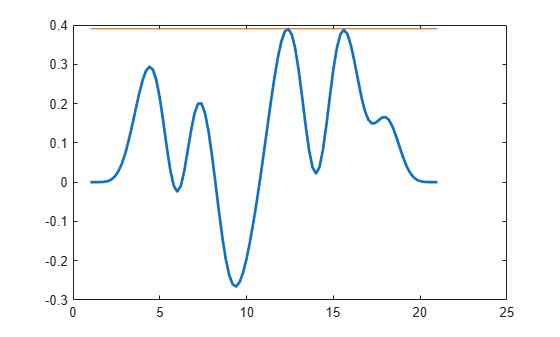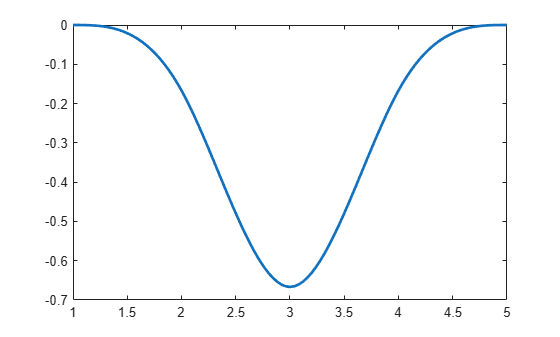fnmin

Minimum of function in given interval

Description

example

fnmin(f) returns the minimum value of the scalar-valued univariate spline in f on its basic interval.

fnmin(f,interv) returns the minimum value of the scalar-valued univariate spline in f on the interval [a..b] specified by interv.

example

[minval,minsite] = fnmin(f,...) also returns a location, minsite, at which the function in f takes that minimum value, minval.

Examples

collapse all

This example shows how to calculate the maximum and minimum values of a spline in f using the fnmin function.

Calculate the Maximum Value

Construct and plot a spline f with 21 knots and 15 random coefficients.

f = spmak(1:21,rand(1,15)-.5);
fnplt(f)

Compute the maximum value of f as the negative of the minimum of -f, then plot it as a horizontal line at the height of the computed maximum.

maxval = -fnmin(fncmb(f,-1));
hold on, plot(fnbrk(f,'interv'),maxval([1 1])), hold offCalculate the Minimum Value

Construct and plot a spline using the spmak function.

f2 = spmak(1:5,-1);
fnplt(f2)Compute the minimum value of f2 and the site at which the spline takes on this minimum value.

[y,x] = fnmin(spmak(1:5,-1))
y = -0.6667
x = 3

Input Arguments

collapse all

Structure of a spline with the fields:

Form of the spline, returned as char.

Non-decreasing sequence of the knots of the spline, returned as a vector.

Coefficients of the spline, returned as a scalar, vector, matrix.

Number of pieces of the spline, returned as a scalar.

Order of the spline, returned as a scalar.

Dimension of the coefficients of the spline, returned as a scalar.

Range of values where the function computes the minimum value of f, specified as a vector.

Data Types: single | double

Output Arguments

collapse all

Minimum value of the scalar-valued univariate spline in f, returned as a scalar.

Site at which the spline in f takes on the minimum value, minval, returned as a scalar.

Algorithms

The fnmin algorithm first changes the basic interval of the function to the given interval, if any. On the interval, fnmin then finds all local extrema of the function as left and right limits at a jump and as zeros of the function's first derivative. It then evaluates the function at these extrema and at the endpoints of the interval, and determines the minimum over all these values.